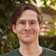# Leetcode - Episode 12 - Starting Early (3 x E)

January 12, 2019

Today we’re doing ‘Saturday Morning Coffee’ solutions — instead of ‘10pm Work Night’ solutions!

Continuing with the streak of string-based problems, all of the code here is ‘optimal’ — i.e., ~97th percentile or higher in the rankings.

### 953. Verifying an Alien Dictionary

Problem: Given the `order` of an alien alphabet, are these given alien `words` ordered lexiographically?

I started with a naive solution to this, which was to iterate `order` into a Dictionary for fast character look-up. I then compared the given list against a `sorted()` version of itself. I.e., `return words == sorted(words, key=some lambda)`. I wasn’t happy with the runtime and I was wasting space — `sorted` creates an addtional list in memory. Also, the function being passed to the lambda converted every word to a numerical list — more created space, more complexity.

I then tested using a list of `[None] * 26` to use as a look-up ‘Dictionary’ by converting the ASCII letters to their numerical values but it was actually slower than using a normal Dictionary which was surprising. So, the Dictionary had to stay.

My improvement was to use a compare function, letter by letter, so that no extra space was created. In some cases only the first letter of each word needed to be checked — instead of converting each whole word.

``````class Solution(object):
def isAlienSorted(self, words, order):
"""
:type words: List[str]
:type order: str
:rtype: bool
"""

# fast char-value checking
vals = dict()
for idx, val in enumerate(order):
vals[val] = idx

for i in range(0, len(words)-1):
w1, w2 = words[i], words[i+1]
flag = 0

for j in range(min(len(w1), len(w2))):
if vals[w1[j]] < vals[w2[j]]:
# w1 is winner
flag = 1
break
elif vals[w1[j]] > vals[w2[j]]:
# w2 is winner
return False

# w1 and w2 have equal comparable chars
if flag != 1:
if len(w1) > len(w2):
return False

return True``````

This is one of my fastest and cleanest solutions yet.

`Runtime: 32 ms, faster than 100.00% of Python online submissions for Verifying an Alien Dictionary.`

Runtime complexity: `O(n)`.

Spacetime complexity: Assuming an `order` of 26: `O(1)`.

### 917. Reverse Only Letters

Problem: Reverse the letters in string `S` leaving all other characters in place.

I used a simple ‘two pointer’ solution to this, similar to a version that I used for 345. Reverse Vowels of a String.

``````from string import ascii_letters
class Solution(object):
def reverseOnlyLetters(self, S):
"""
:type S: str
:rtype: str
"""
S = list(S)
letters = set(ascii_letters)
start = 0
end = len(S)-1
while not start > end:
if S[start] not in letters:
start += 1
continue
if S[end] not in letters:
end -= 1
continue
S[start], S[end] = S[end], S[start]
start += 1
end -= 1
return ''.join(S)``````

After researching, and checking the runtime percentile (~97th), I believe this to be the most optimal Python solution to this problem.

Runtime complexity: `O(n)`.

Space complexity: `O(1)`.

### 844. Backspace String Compare

Problem: Given string `S` and `T`, are they equal when written in a text editor when `#` is backspace?

One of my early solutions was creating two lists, e.g., `[None] * len(S)`, but I realised that this wasn’t optimal and may create more space than required.

I reached for `deque` — Python’s implementation of a Double Ended Queue. With backspace equalling a `pop()` the rest was pretty straight forward.

``````from collections import deque
class Solution(object):
def backspaceCompare(self, S, T):
"""
:type S: str
:type T: str
:rtype: bool
"""
s = deque()
for i in S:
if i == '#':
if len(s) > 0:
s.pop()
else:
continue
else:
s.append(i)

t = deque()
for i in T:
if i == '#':
if len(t) > 0:
t.pop()
else:
continue
else:
t.append(i)

return s == t``````

I saw a faster solution to this problem that doesn’t use any data structures. Instead it uses while loops and a lot of checking. It’s about twice as many lines of code as mine and, while impressive, is incredibly hard to parse!

Runtime complexity: `O(n)`.

Spacetime complexity: `O(n)`.